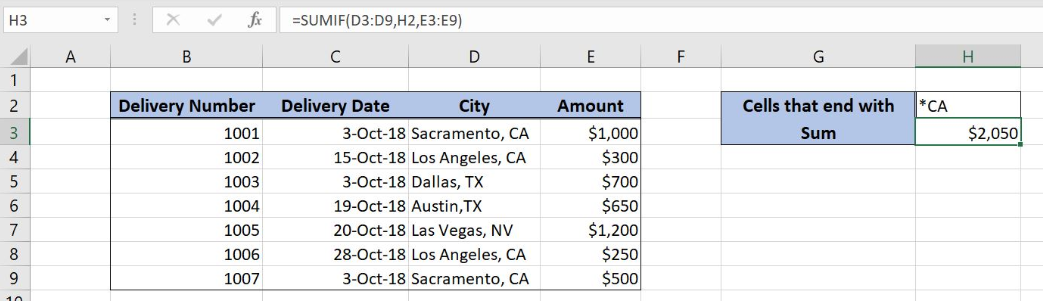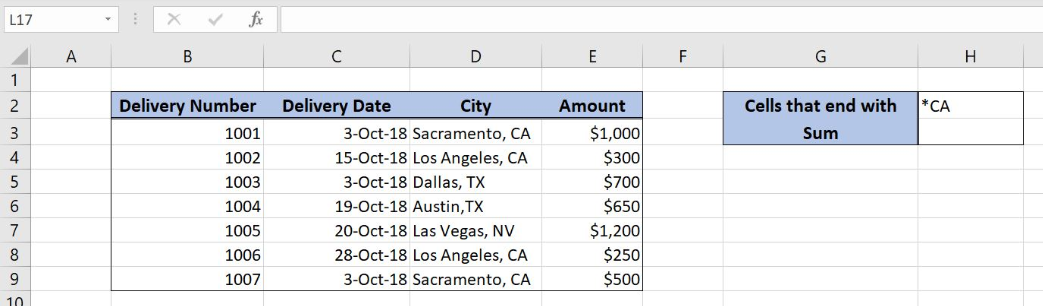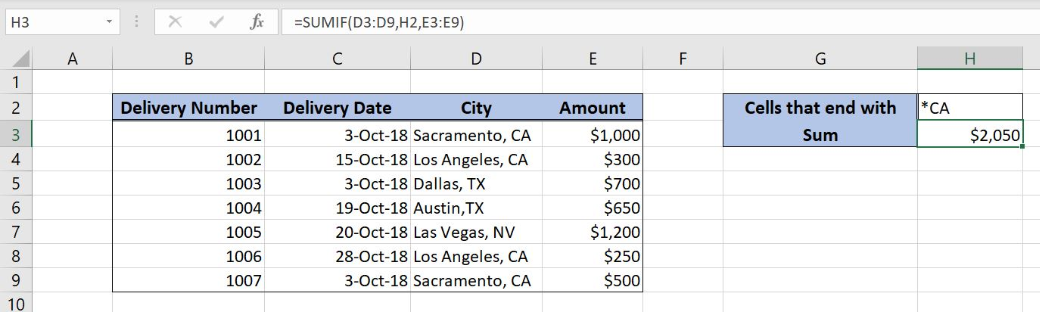Get instant live expert help with Excel or Google Sheets“My Excelchat expert helped me in less than 20 minutes, saving me what would have been 5 hours of work!”

#### Post your problem and you’ll get Expert help in seconds.

Your message must be at least 40 characters
Our professional Expert are available now. Your privacy is guaranteed.

# Sum If Ends WithRead time: 15 minutes

Excel allows a user to sum cells that end with certain characters, by using the SUMIF function. This step by step tutorial will assist all levels of Excel users in summing cells that end with selected text.Figure 1. The result of the SUMIF function

## Syntax of the SUMIF Formula

The generic formula for the SUMIF function is:

`=SUMIF(range, criteria, [sum_range])`

The parameters of the SUMIF function are:

• range – a range where we want to apply our criteria
• criteria – a criteria which we want to apply to a range
• [sum_range] – a range where we want to sum. This is an optional parameter. If we omit it, the function will sum amounts from a range parameter.

## Setting up Our Data for the SUMIF FunctionFigure 2. Data that we will use in the SUMIF example

In the data table, we have 4 columns:  “Delivery Number” (column B), “Delivery Date” (column C), “City” (column D) and “Amount” (column E). In the cell H2, we have a criteria for the function, while in H3 we want to get a sum.

## Count Cells That End With a Certain Text

In our example, we want to sum all values from column E, for which value in column D ends with “CA”.

The formula looks like:

`=SUMIF(D3:D9, H2, E3:E9)`

The parameter range is D3:D9 and the criteria is in the cell H2. The sum_range is E3:E9. We have to put an asterisk in criteria (*CA). An asterisk stands for any character.

To apply the SUMIF function, we need to follow these steps:

• Select cell H3 and click on it
• Insert the formula: `=SUMIF(D3:D9, H2, E3:E9)`
• Press enterFigure 3. Counting the cells that begins with a certain text

As you can see, cells D3, D4, D8 and D9 end with “CA”, so the result of the function in the cell H3 is \$2,050.

Most of the time, the problem you will need to solve will be more complex than a simple application of a formula or function. If you want to save hours of research and frustration, try our live Excelchat service! Our Excel Experts are available 24/7 to answer any Excel question you may have. We guarantee a connection within 30 seconds and a customized solution within 20 minutes.

### Did this post not answer your question? Get a solution from connecting with the expert.Another blog reader asked this question today on Excelchat:## Subscribe to Excelchat.coAnother blog reader asked this question today on Excelchat: